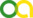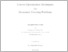Rolfes, Jan Hendrik (2019). Convex Optimization Techniques for Geometric Covering Problems. PhD thesis, Universität zu Köln.Preview
PDF
DissertationRolfes.pdf - Accepted Version

## Abstract

The present thesis is a commencement of a generalization of covering results in specific settings, such as the Euclidean space or the sphere, to arbitrary compact metric spaces. In particular we consider coverings of compact metric spaces $(X,d)$ by balls of radius $r$. We are interested in the minimum number of such balls needed to cover $X$, denoted by $\Ncal(X,r)$. For finite $X$ this problem coincides with an instance of the combinatorial \textsc{set cover} problem, which is $\mathrm{NP}$-complete. We illustrate approximation techniques based on the moment method of Lasserre for finite graphs and generalize these techniques to compact metric spaces $X$ to obtain upper and lower bounds for $\Ncal(X,r)$. \\ The upper bounds in this thesis follow from the application of a greedy algorithm on the space $X$. Its approximation quality is obtained by a generalization of the analysis of Chv\'atal's algorithm for the weighted case of \textsc{set cover}. We apply this greedy algorithm to the spherical case $X=S^n$ and retrieve the best non-asymptotic bound of B\"or\"oczky and Wintsche. Additionally, the algorithm can be used to determine coverings of Euclidean space with arbitrary measurable objects having non-empty interior. The quality of these coverings slightly improves a bound of Nasz\'odi. \\ For the lower bounds we develop a sequence of bounds $\Ncal^t(X,r)$ that converge after finitely (say $\alpha\in\N$) many steps: $$\Ncal^1(X,r)\leq \ldots \leq \Ncal^\alpha(X,r)=\Ncal(X,r).$$ The drawback of this sequence is that the bounds $\Ncal^t(X,r)$ are increasingly difficult to compute, since they are the objective values of infinite-dimensional conic programs whose number of constraints and dimension of underlying cones grow accordingly to $t$. We show that these programs satisfy strong duality and derive a finite dimensional semidefinite program to approximate $\Ncal^2(S^2,r)$ to arbitrary precision. Our results rely in part on the moment methods developed by de Laat and Vallentin for the packing problem on topological packing graphs. However, in the covering problem we have to deal with two types of constraints instead of one type as in packing problems and consequently additional work is required.

Item Type: Thesis (PhD thesis)
Translated abstract:
AbstractLanguage
Die vorliegende Arbeit ist der Beginn einer Verallgemeinerung von Resultaten über spezifische Überdeckungen, wie etwa Überdeckungen des euklidischen Raumes oder Überdeckungen der Sphäre, zu einer Theorie auf kompakten metrischen Räumen. Insbesondere betrachtet man Überdeckungen eines kompakten metrischen Raumes $(X,d)$ durch Kugeln mit Radius $r$. Das Augenmerk liegt hierbei auf der minimalen Anzahl solcher Kugeln, welche benötigt wird um $X$ zu überdecken. Wir bezeichnen diese Anzahl mit $\Ncal(X,r)$. Für endliche Räume $X$ entspricht dieses Problem einer Instanz des kombinatorischen \textsc{set cover} Problems, welches NP-vollständig ist. Wir beschreiben Approximationstechniken, basierend auf der Momentenmethode von Lasserre für endliche Graphen und verallgemeinern diese Techniken auf kompakte metrische Räume um untere und obere Schranken zu erhalten. \\ Die oberen Schranken in dieser Arbeit folgen aus der Anwendung eines Greedy-Algorithmus auf den Raum $X$. Die Approximationsgüte des Algorithmus erhalten wir durch eine Verallgemeinerung der Analyse von Chv\'atals Algorithmus für gewichtete \textsc{set cover} Probleme. Wir wenden den genannten Greedy-Algorithmus auf den sphärischen Fall $X=S^n$ an und erhalten die beste, nicht asymptotische Schranke von Böröczky und Wintsche. Weiterhin kann der Algorithmus genutzt werden, um Überdeckungen des euklidischen Raumes durch beliebige messbare Objekte mit nicht leerem Inneren zu bestimmen. Die Approximationsgüte dieser Überdeckungen stellt eine leichte Verbesserung der Schranken von Nasz\'odi dar. \\ Um untere Schranken zu erhalten entwickeln wir eine Folge von Schranken $\Ncal^t(X,r)$, welche nach endlich vielen (bezeichnet mit $\alpha\in\N$) Schritten konvergiert: $$\Ncal^1(X,r)\leq \ldots \leq \Ncal^\alpha(X,r)=\Ncal(X,r).$$ Der Nachteil dieser Folge ist, dass die Schranken $\Ncal^t(X,r)$ mit wachsendem $t$ immer schwieriger zu berechnen sind, da sie die Zielfunktionswerte unendlichdimensionaler konischer Programme sind, deren Anzahl an Bedingungen und Dimension der Kegel mit $t$ wachsen. Wir zeigen, dass diese Programme die Bedingung der starken Dualität erfüllen und leiten ein endlichdimensionales semidefinites Programm ab, welches darauf abzielt $\Ncal^2(S^2,r)$ in beliebiger Präzision zu approximieren. Unsere Ergebnisse basieren teilweise auf der Momentenmethode von de Laat und Vallentin für das Packungsproblem auf topologischen Packungsgraphen. Jedoch müssen wir uns im Überdeckungsproblem um zwei Arten von Bedingungen kümmern anstatt nur einer Art wie im Packungsproblem. Dies benötigt zusätzlichen Aufwand.German
Creators:
CreatorsEmailORCIDORCID Put Code
Rolfes, Jan HendrikJ_Rolfes@web.deUNSPECIFIEDUNSPECIFIED
URN: urn:nbn:de:hbz:38-99176
Date: 28 March 2019
Language: English
Faculty: Faculty of Mathematics and Natural Sciences
Divisions: Faculty of Mathematics and Natural Sciences > Department of Mathematics and Computer Science > Mathematical Institute
Subjects: Data processing Computer science
Mathematics
Uncontrolled Keywords:
KeywordsLanguage
geometric covering, metric entropy, polynomial optimization, set cover, greedy algorithm, moment methodEnglish
Geometrische Überdeckungen, Metrische Entropie, polynomielle Optimierung, Mengenüberdeckungsproblem, Greedy Algorithmus, MomentenmethodeGerman
Date of oral exam: 28 March 2019
Referee:
Vallentin, FrankProf. Dr.
Gross, DavidProf. Dr.
Riener, CordianProf. Dr.
Refereed: Yes
URI: http://kups.ub.uni-koeln.de/id/eprint/9917View Item